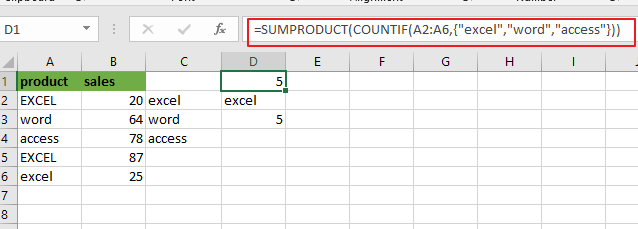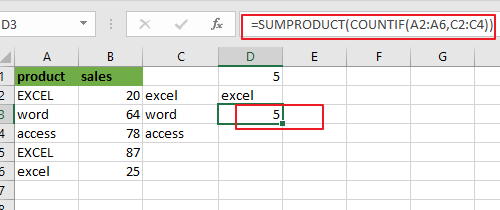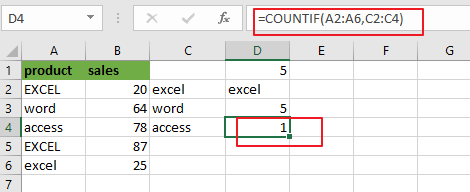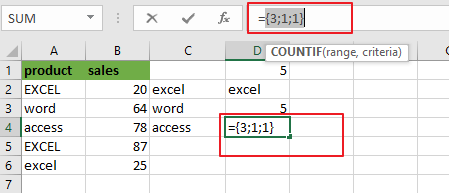# How to Count Cells equal to one of many cells in Excel

This post will guide you how to count the number of cells equal to many things in a given range cells using a formula in Excel 2013/2016.You can easily to count cells equal to a specific value through COUNTIF function. But if there is an easy way to count cells equal to one of many values defined in a selected range of cells in Microsoft Excel. The SUMPRODUCT function can be used to check if a cell is equal to one or many things from a given range. And TRUE or FALSE values will be returned.

## Count Cells equal to one of many cells

Assuming that you want to count cells that contain value “EXCEL”, ”access” or “word” defined in a selected range(A1:B6).In this case, you can use the COUNTIF function in combination with the SUMPRODUCT function to count the number of cells equal to one or many things. Using the following formula:

=SUMPRODUCT(COUNTIF(A2:A6,{“excel”,”word”,”access”}))

Or

=SUMPRODUCT(COUNTIF(A2:A6,C2:C4))Note: for the above formula, A2:A6 is a range of cells that you want to use, and C2:C4 or {“excel”,”word”,”access”}is the values that you wish to count. You also need to know that the COUNTIF function is not case-sensitive.

Now Let’s see how this formula works:

The COUNTIF function will count the number of cells in a given range that match supplied criteria. In the above formula, multiple values were passed into COUNTIF function as its arguments, it should return an array of numbers as its result, where each number indicates the count of one value in range A2:A6. Since range C2:C4 contain three values or array list contain three values (“excel”, “word” and “access”), so the COUNTIF function will return 3 result in an array, like below:

=COUNTIF(A2:A6,C2:C4)={3;1;1}Note: you need to know that the COUNTIF function is not case sensitive. And if you want to count the number of cells in a range with case-sensitive, you need to use EXACT function.

### Related Functions

• Excel SUMPRODUCT function
The Excel SUMPRODUCT function multiplies corresponding components in the given one or more arrays or ranges, and returns the sum of those products. The syntax of the SUMPRODUCT function is as below:= SUMPRODUCT (array1,[array2],…)…
• Excel COUNTIF function
The Excel COUNTIF function will count the number of cells in a range that meet a given criteria. This function can be used to count the different kinds of cells with number, date, text values, blank, non-blanks, or containing specific characters.etc.= COUNTIF (range, criteria)…
• Excel EXACT function
The Excel EXACT function compares if two text strings are the same and returns TRUE if they are the same, Or, it will return FALSE.The syntax of the EXACT function is as below:= EXACT (text1,text2)…